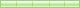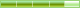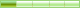xueli521macro_command main()

bool a,b,c = 1 ,d = 0
int e

GetData(a,"HMI",LB,2,1)
GetData(b,"HMI",LB,3,1)

while (a == true and b == false)
SetData(c,"HMI",LB,0,1)
SetData(d,"HMI",LB,1,1)
DELAY(1000)
SetData(d,"HMI",LB,0,1)
SetData(C,"HMI",LB,1,1)
DELAY(1000)
if b == true then
break
end if
wend
end macro_command
fjqz001楼  发表于: 19天前2楼  发表于: 18天前

while (a == true and b == false)
~~~
GetData(a,"HMI",LB,2,1)
GetData(b,"HMI",LB,3,1)
~~~
wend
ren18233楼  发表于: 18天前

xueli5214楼  发表于: 14天前